•  总之，队列中的点都是当前有可能作为起点的，是一条下凸曲线。这样就比遍历一遍省时间，省去了一些没用的点。  这个题还是要画图才看得清楚。。 #include #include #include #include #include #...

A DNA sequence consists of four letters, A, C, G, and T. The GC-ratio of a DNA sequence is the number of Cs and Gs of the sequence divided by the length of the sequence. GC-ratio is important in gene finding because DNA sequences with relatively high GC-ratios
might be good candidates for the starting parts of genes. Given a very long DNA sequence, researchers are usually interested in locating a subsequence whose GC-ratio is maximum over all subsequences of the sequence. Since short subsequences with high GC-ratios
are sometimes meaningless in gene finding, a length lower bound is given to ensure that a long subsequence with high GC-ratio could be found. If, in a DNA sequence, a 0 is assigned to every A and T and a 1 to every C and G, the DNA sequence is transformed
into a binary sequence of the same length. GC-ratios in the DNA sequence are now equivalent to averages in the binary sequence.

Position

1
1
1
1
1
1
1
1
Index
1
2
3
4
5
6
7
8
9
0
1
2
3
4
5
6
7
Sequence
0
0
1
0
1
0
1
1
0
1
1
0
1
1
0
1
0

For the binary sequence above, if the length lower bound is 7, the maximum average is 6/8 which happens in the subsequence [7,14]. Its length is 8, which is greater than the length lower bound 7. If the length lower bound is 5, then the subsequence [7,11] gives
the maximum average 4/5. The length is 5 which is equal to the length lower bound. For the subsequence [7,11], 7 is its starting index and 11 is its ending index.
Given a binary sequence and a length lower bound L, write a program to find a subsequence of the binary sequence whose length is at leastL and whose average is maximum over all subsequences
of the binary sequence. If two or more subsequences have the maximum average, then find the shortest one; and if two or more shortest subsequences with the maximum average exist, then find the one with the smallest starting index.
Input
Your program is to read from standard input. The input consists of
T test cases. The number of test cases T is given in the first line of the input. Each test case starts with a line containing two integersn(1n100,
000) andL (1L1,
000) which are the length of a binary sequence and a length lower bound, respectively. In the next line, a string, binary sequence, of lengthn is given.
Output
Your program is to write to standard output. Print the starting and ending index of the subsequence.
The following shows sample input and output for two test cases.
Sample Input
2
17 5
00101011011011010
20 4
11100111100111110000

Sample Output
7 11
6 9

找一个长度不小于L的子串让里面1的比例最大，如果有多个，找长度最短的，如果还有多个，找最靠前的。
我也是想到用sum存前面的数字之和，找最大的(sum[b]-sum[a])/(b-a)。可是没想出来怎么找a，遍历一遍肯定是不行的会超时。。还是在网上搜了做法，以前没见过这样的。
这相当于一个非递减数列，i是x坐标，sum[i]是y坐标，在这个图上找y-x>=L的斜率最大的两个点。用一个队列保存当前可能用到的起点，设i从L递增到N，我们每次在队列中加入i-L作为起点，设rear为当前队列中最后一个元素。如果发现rear和i-L这两个点的斜率小于等于rear-1和rear这两个点的斜率，也就是rear-1,rear,i-L三个点一次连成的曲线不是下凸的，就可以把rear这个点删掉了。这是因为后面再出现的点怎么都不可能和rear这个点构成最大斜率（可以自己画个图看一下）。从后删到不能删后，把i-L加到最后。不光可以从后删掉一些没用的点，还可以从前删掉一些。如果front那个点和i点的斜率小于front+1和i的斜率，那么front这个点可以删掉，因为y是单调不减的，后面再出现的点如果可能更新最大斜率的话，明显和front+1构成的斜率更优。这样又从前删掉了一些点。删完后i和当前的front就是i点作为end的最优答案。
总之，队列中的点都是当前有可能作为起点的，是一条下凸曲线。这样就比遍历一遍省时间，省去了一些没用的点。
这个题还是要画图才看得清楚。。

#include<cstdio>
#include<algorithm>
#include<iostream>
#include<sstream>
#include<cstring>
#include<cmath>
#include<queue>
#include<map>
#include<set>
#define INF 0x3f3f3f3f
#define MAXN 100010
#define MAXM 1010
#define eps 1e-9
#define pii pair<int,int>
using namespace std;
int T,N,L;
int q[MAXN],sum[MAXN];
char str[MAXN];
double k(int a,int b){
return (double)(sum[b]-sum[a])/(b-a);
}
int main(){
freopen("in.txt","r",stdin);
scanf("%d",&T);
while(T--){
scanf("%d%d%s",&N,&L,str);
sum=0;
for(int i=1;i<=N;i++) sum[i]=sum[i-1]+str[i-1]-'0';
int front=0,rear=-1,len=0,start=0,end=L;
double ans=0;
for(int i=L;i<=N;i++){
int s=i-L;
while(front<rear&&k(q[rear],s)<=k(q[rear-1],q[rear])) rear--;
q[++rear]=s;
while(front<rear&&k(q[front],i)<=k(q[front+1],i)) front++;
double m=k(q[front],i);
int l=i-q[front]+1;
if(m==ans&&l<len||m>ans){
ans=m;
len=l;
start=q[front];
end=i;
}
}
printf("%d %d\n",start+1,end);
}
return 0;
}


展开全文• 本文给出了沿R"中凸曲线的极大函数算子与Hilbert变换具有L0(1
• 数学里上凹，凹，上下凸统称为曲线知性，其是指在平面坐标系里的图形样式： 1、开口向上的曲线，称为上凹，或称为下凸，形状为 ∪； 2、开口向曲线，称为凹，或称为上，形状为 ∩； 3、...
https://zhidao.baidu.com/question/238541854.html

数学里上凹，下凹，上凸，下凸统称为曲线的凸知性，其是指在平面坐标系里的图形样式：

1、开口向上的曲线，称为上凹，或称为下凸，形状为 ∪；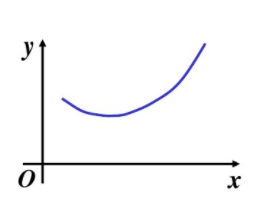2、开口向下的曲线，称为下凹，或称为上凸，形状为 ∩；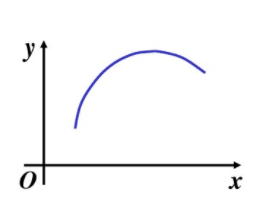3、所以上凹，下凹，上凸，下凸四种，实际上可归类为上凸，下凸两道种情况：

（1）从切线角度讲，下凸弧上过任一点的切线都在曲线弧之下，而上凸弧上过任一点的切线都在曲线弧之上。

（2）从割线角度讲，如果连续曲线y=f(x)在区间(a，b)对应内的曲线弧上任意两点的割线线段都在该两点间的曲线弧之上，则称该段曲线弧是下凸的，并称函数y=f(x)在区间(a，b)上是下凸的(或上凹的，即曲线开口向上)。反之，则是上凸的。

（3）从导数角度讲，设y=f(x)在(a，b)内容具有二阶导数，如果在(a，b)内f''(x)>o，则y=f(x)在(a，b)内为下凸；如果在(a，b)内f''(x)<o，则y=f(x)在(a，b)内为上凸。

展开全文• In this paper the methods generating contiguous Bezier two-gons and three-gons are given. So that we construct the convex interpolate spline curves which pass data-points and are shape-preserving and ...
•研究论文
• 构造了一种分母为二次的有理三次插值函数。它是C连续的。在给定的插值数据条件,通过调整插值函数中的参数,给出了插值曲线的保方法和该方法得以实现的条件。
• 给定平面上一列数据点，导出了用具有一阶几何连续性的分段二次多项式参数曲线插值各型值点且具有保凸性的充分必要条件。并用一些实例进行验证。结果表明，这种方法是正确和有效的。
• 贝塞尔曲线插值与B样条插值 前言： 这篇是“样条曲线”的接续，前面主要集中在了理论部分，这篇文章主要内容是贝塞尔曲线与B样条是如何应用到插值中的。 前篇：样条曲线 文章目录贝塞尔曲线插值与B样条插值0. 插值...
贝塞尔曲线插值与B样条插值

前言： 这篇是“样条曲线”的接续，前面主要集中在了理论部分，这篇文章主要内容是贝塞尔曲线与B样条是如何应用到插值中的。
前篇：样条曲线

文章目录贝塞尔曲线插值与B样条插值0. 插值问题1. 贝塞尔曲线插值1.1 曲线的数学描述1.2 曲线插值1.3  代码实现2. B样条插值2.1 数学表达和一些补充2.2 曲线插值2.2.1 三次 clamped B-样条2.2.2 B样条插值方程的获取2.2.3 方程求解，确定样条曲线3. 代码实现（挖坑）3. 三次样条插值4. 总结
0. 插值问题
问题是一切发展的动力。还是从问题出发，考虑怎么通过数学手段解决问题。
对于插值问题，就是已知一些离散点，插值出一些新的点，使得所有点形成一条光滑曲线。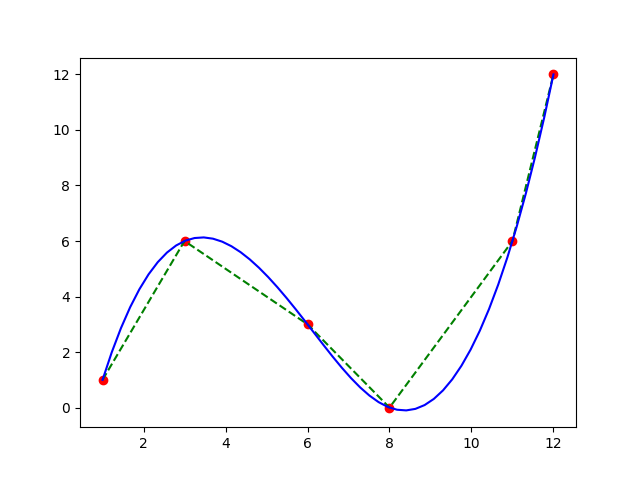如已知六个点 $P_0,P_1,\cdots,P_5$ ，如图红色点 $(1,1),(3,6),(6,3),(8,0),(11,6),(12,12)$ ，如何通过插值生成类似于图中蓝色光滑曲线，而非僵硬的绿色多线段。
1. 贝塞尔曲线插值
1.1 曲线的数学描述
依据前篇的数学描述，假设一共 $n+1$ 个控制点 $P_0,P_1,\cdots,P_n$ ，这 $n+1$ 个点可以确定一条 $n$  次贝塞尔曲线。可以用如下式计算曲线上任意点坐标
$B(t) = \sum\limits_{i=0}^n C_n^i(1-t)^{n-i}t^i P_i$
特别的，有几个常用贝塞尔曲线
二次贝塞尔曲线
$B(t) = (1-t)^2P_0 + 2t(1-t)P_1 + t^2 P_2, \ \ \ \ \ t\in[0,1]$
三次贝塞尔曲线
$B(t) = (1-t)^3P_0 + 3t(1-t)^2P_1 + 3t^2(1-t)P_2 + t^3P_3$
1.2 曲线插值
这里以最常用的三次贝塞尔曲线为例，探究其插值应用。
对于贝塞尔曲线，有三个要点：

其是通过控制点来生成的，控制点不全在最终曲线上。
控制点首末的两点是最终曲线的端点（意味着首末控制点会在最终曲线上），且各自与相邻点的连线同最终曲线相切。
两个贝塞尔曲线如果平滑连接，则需要连接点与其左右相邻两端点共线。

基于要点2，可以在把两个已知点作为三次贝塞尔曲线的两个端点 $P_0,P_3$，然后想办法再指定两个控制点 $P_2,P_3$ 即可。
且控制点设定要满足贝塞尔曲线相连时平滑。
总结一下就是：

在两点中生成贝塞尔曲线，且这两个点作为三次贝塞尔曲线的两个端点。
再确定额外两个控制点，控制点设定满足两相邻的贝塞尔曲线连接点和其连接点左右控制点共线。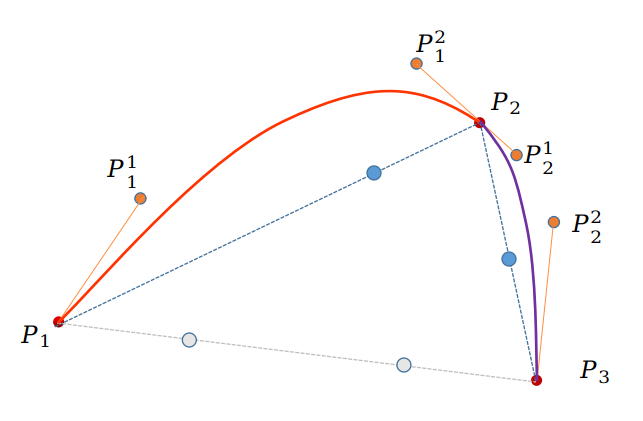如上图是由两个贝塞尔曲线组成的一条平滑曲线，经过点 $P_1,P_2,P_3$ ，且 $P_1,P_2$ 是第一条三次贝塞尔曲线的两端点，$P_2,P_3$ 是第二条三次贝塞尔曲线的两端点。我们需要的是每个贝塞尔曲线再设定两个控制点即可。
最简单直接的思路就是列方程，解方程。如上，两条贝塞尔曲线，一共四个未知点(控制点)，需要四个方程。

令其连接点满足 $c^1$ 连续，也即 $P_1^2,P_2,P_2^1$ 共线。此处有一个方程。

对连接点处 $c^2$ 连续也加以考虑。此处也有一个方程。
还差两个方程。限制两端点即可。比如二阶导为某一定值，或三阶导为某一定值。诸如此类，这一点在后面会有详细描述。B样条中，简单的三次样条中都有详细描述。

这种思路原理直接且简单，不再用代码实现。无非就是列方程、解方程的问题。这一点在B样条中有详细类似的过程，但比这复杂的多。
下面详细描述一种有趣的解决方案：
最重要的一点是满足 $P_1^2,P_2,P_2^1$ 三点共线，所以只要关注$P_1^2,P_2^1$ 的设定即可，因为$P_1^1,P_2^2$ 可以依据同样的规则设定。解决方案如下，$P_1^2,P_2^1$ 肯定不是凭空产生，需要依赖于已有的一些点。而其中可依赖的点有 $P_1,P_2,P_3$ 。
第一种思路可以在$P_1,P_2$ 连线上，控制一个比例因子生成一点，同样 $P_2,P_3$ 连线上亦如是。如上图中的蓝色两点，然后连接两点形成一条线段，平移线段，使其通过 $P_2$ ，平移后的两点就是我们需要的 $P_1^2,P_2^1$ 。
第二种思路可以直接在$P_1,P_3$ 连线上直接根据某特定比例因此生成两点，按照同样的平移步骤，平移后的该两点当作控制点。当然比例因此可以根据先验信息，比如参考线 $P_1P_2$ 和线 $P_2,P_3$ 长度比的数值。等等。
1.3  代码实现
import numpy as np
import matplotlib.pyplot as plt

def getInterpolationPoints(controlPoints, tList):
n = len(controlPoints)-1
interPoints = []
for t in tList:
Bt = np.zeros(2, np.float64)
for i in range(len(controlPoints)):
Bt = Bt + comb(n,i) * np.power(1-t,n-i) * np.power(t,i) * np.array(controlPoints[i])
interPoints.append(list(Bt))

return interPoints

def getControlPointList(pointsArray, k1=-1, k2=1):
points = np.array(pointsArray)
index = points.shape - 2

res = []
for i in range(index):
tmp = points[i:i+3]
p1 = tmp
p2 = tmp
p3 = tmp

if k1 == -1:
l1 = np.sqrt(np.sum((p1-p2)**2))
l2 = np.sqrt(np.sum((p2-p3)**2))
k1 = l1/(l1+l2)
k2 = l2/(l1+l2)

p01 = k1*p1 + (1-k1)*p2
p02 = (1-k2)*p2 + k2*p3
p00 = k2*p01 + (1-k2)*p02

sub = p2 - p00
p12 = p01 + sub
p21 = p02 + sub

res.append(p12)
res.append(p21)
pFirst = points + 0.1*(res - points)
pEnd = points[-1] + 0.1*(res[-1] - points[-1])
res.insert(0,pFirst)
res.append(pEnd)

return np.array(res)

if __name__ == '__main__':
points = [[1,1],[3,6],[6,3],[8,0],[11,6],[12,12]]
controlP = getControlPointList(points)
l = len(points) - 1
figure = plt.figure()
t = np.linspace(0,1,50)
for i in range(l):
p = np.array([points[i], controlP[2*i], controlP[2*i+1], points[i+1]])
interPoints = getInterpolationPoints(p, t)
x = np.array(interPoints)[:,0]
y = np.array(interPoints)[:,1]
plt.plot(x,y)
plt.scatter(np.array(points)[:,0],np.array(points)[:,1],color='gray')
plt.show()

结果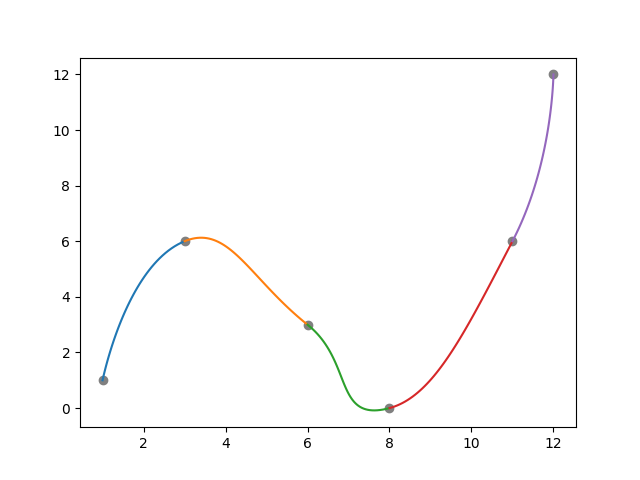分析
结果如预期一样，不会特别好，但可以确定该种方法是可行的。结果受比例因子 $k1,k2$ 影响很大，受原始点（型值点）的密度、趋势变化影响。
观察也知，该想法对于没有明显趋势反转（凸曲线变为凹曲线）的点效果还行，但对于转折点，效果不太行。分析其原因，是在起初分析时，使用的图是便没有考虑这种情况。如下，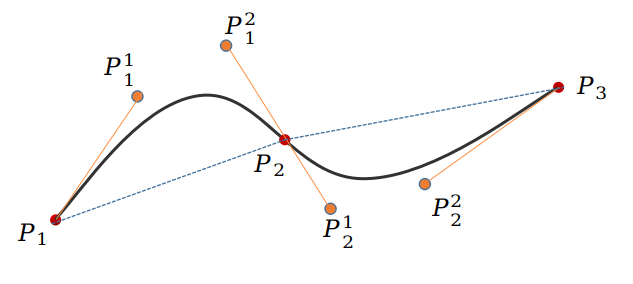而该问题的本质原因，是因为该思路只限制了连接点处 $c^1$ 连续，而对$c^2$ 连续没有考虑，所以就出现了结果图中连接点确实看起来很平滑，但后面的过渡不太好。这也侧面说明了插值问题中 $c^2$ 连续对于平滑是很重要的。
2. B样条插值
2.1 数学表达和一些补充
具体推导见前篇：样条曲线
对于 n+1 个控制点 $P_0,P_1,\cdots,P_n$ ，有一个包含 m+1 个节点的列表（或节点向量）${t_0,t_1,\cdots,t_{m}}$ ，其 k 次B样条曲线表达式为（且m=n+k+1）
$P(t) = \sum\limits_{i=0}^n B_{i,k}(t)\ P_i$
其中 $B_{i,k}(t)$ 称为 k 次 B 样条基函数，也叫调和函数。且 $B_{i,k}(t)$ 满足如下递推式（de Boor递推式）
$k = 0,\ \ \ \ B_{i,0}(t) = \left\{ \begin{matrix} 0, \ \ \ \ t\in[t_i,t_i+1] \\ 1, \ \ \ \ \ \ \ \ Otherwise \end{matrix} \right.\\ k > 0,\ \ \ \ B_{i,k}(t) = \frac{t-t_i}{t_{i+k}-t_i} B_{i,k-1}(t) + \frac{t_{i+k+1}-t}{t_{i+k+1}-t_{i+1}} B_{i+1,k-1}(t)$

前篇中在尝试各种节点列表时，得到了很多结果，但对结果总结分析较少，以下是一些非常重要的小总结和补充

前篇实例中许多曲线看起来很奇怪，是因为没有考虑定义域，也即由基函数完全支持的部分。该定义域在前篇中有提到，是 $[t_k,t_{n+1}]\ \ or\ \ [t_k,t_{m-k}]$ ，这点十分重要。在考虑定义域的情况下，曲线平滑且不会显示地很奇怪。
定义域是依赖于节点列表的，值得一提的是，如果想要较广定义域$[t_k,t_{n+1}]$，从式子上来看，至少有两种思路

使得定义域内 t 区间数量尽量多，这种情况在节点均匀分布时可用，别的情况可能没用。即 $k$ 尽量小，$n+1$ 尽量大，也即 $m-(n+1)$ 尽量小，$n+1$ 尽量大。也即 $m$ 与 n+1 尽量接近，n+1 尽量大。换句话说，节点个数尽量多，控制点数与节点数接近。
使用该方法重新设定节点列表绘制前篇中第一个实例曲线
$controlPonts = [[50,50], [100,300], [300,100], [380,200], [400,600]]\\ knots = \{0,\frac{1}{20},\frac{2}{20},\frac{8}{20},\frac{14}{20},\frac{19}{20},1,1\} \\ n=4,m=7,k=2 \\ domain = [t_3,t_5] = [\frac{2}{20},\frac{19}{20}]$
定义域已经几乎包括了所有区间，最终定义域内曲线如下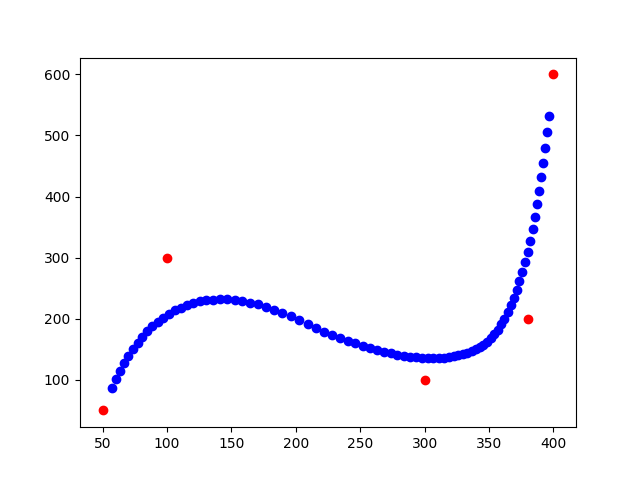曲线没有包含全部，但 $[\frac{2}{20},\frac{19}{20}]$ 也几乎包含全部了。Excellent！

使得定义域内 t 区间跨度大，其实严格来说就是我们要解决的问题本质，第一种思路只是实现第二种的一种解决方案。跨度大，也即 $t_{n+1} - t_k$ 尽量大。如果 $m,n,k$ 确定的情况下，如何确保呢。可以改变节点列表中的 t 的分布。我们需要的是区间$[t_k,t_{m-k}]$ ，如果前 k+1 个 t 数据全部设为 0，而第 m-k 个 t 之后的数据全部设为 1。那么区间 $[t_k,t_{m-k}]$ 跨度不就是 $[0,1]$ 嘛，也就是整个曲线嘛，很棒的想法。从曲线的角度来看，此时就是我们绘制出的整个曲线。就没有之前绘图中出现的奇奇怪怪的曲线变化了。
使用该方法重新设定节点列表绘制前篇中第一个实例曲线
$controlPonts = [[50,50], [100,300], [300,100], [380,200], [400,600]]\\ knots = \{0,0,0,0,\frac{1}{2},1,1,1,1\} \\ n=4,m=8,k=3 \\ domain = [0,1]$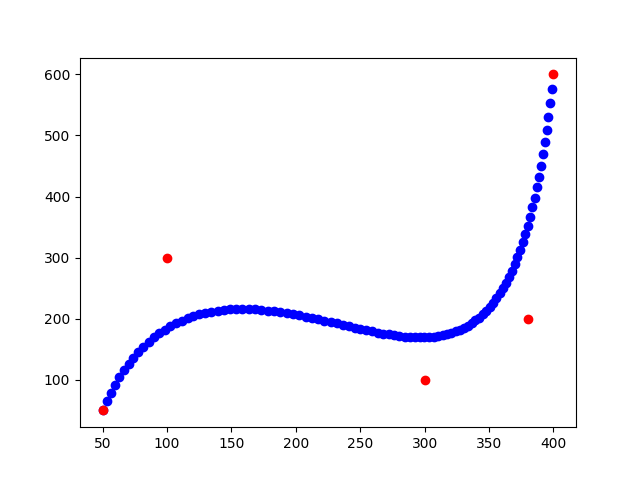不再有奇怪的点，生成的曲线也十分"完美"。

第二种思路极其重要，如果 $[t_k,t_{m-k}]$ 区间内节点又均匀分布，该样条曲线也被称作为准均匀样条。在首末两端设定一定数量的 0,1 的方法在样条曲线中极其常见，因为它确实很实用啊。

前篇中也提到了一个有趣且及其重要的现象，即 当节点列表中首末两端 0,1 重复 k+1次时，那么生成的样条曲线首末两端同贝塞尔曲线一样，都是实际原始点（型值点）的首末两点。该样条曲线也有个特定的名字，叫 clamped B-spline curves 。结合上面第二种思路提到的内容，我们得到的样条曲线也就是整条曲线。

其中还有一个 Wrapping 问题，即，使得生成曲线形成闭环。待用到时再探究和总结（挖坑）。

2.2 曲线插值
贝塞尔曲线中应用插值时提到了生成曲线端点为数据点（型值点）的重要性，该特点是实现插值的基础，或者说是实现两点间插值的基础。幸运的是，B样条中有一种样条满足这样的条件，即上述的 clamped B-spline。需要节点首末的 $0,1$ 重复$k+1$ 次。
我们常用三次的样条插值，下面就以三次样条插值为例，对其进行详细分析。
2.2.1 三次 clamped B-样条
对于三次 camped B-样条，有以下要点

次数 k
$k = 3$

型值点个数 $n+1$ 与 节点个数 $m+1$ 满足

$m = n + k + 1\ \ \Rightarrow\ \ m - n = 4$

camped 情况下，节点 $t_0,t_1,\cdots,t_m$ 满足
\left\{ \begin{matrix} \begin{aligned} &t_0 = t_1 = t_2 = t_3 = 0 \\ &t_4,t_5,\cdots,t_{n} \in [0,1] \\ &t_{n+1} = t_{n+2} = t_{n+3} = t_{n+4} = 1 \end{aligned} \end{matrix} \right.
特别地，如果使用准均匀样条，即 $t_4,t_5,\cdots,t_n$ 均匀分布（当然也可以使用别的分布方法），即有
\begin{aligned} &t_4 = \frac{4-3}{n+1-k} = \frac{1}{n-2}\\ &t_5 = \frac{5-3}{n-2} = \frac{2}{n-2} \\ &\cdots \\ &t_j = \frac{j-3}{n-2} \\ &\cdots \\ &t_n = \frac{n-3}{n-2} \end{aligned}

为了形象展示，使用之前类似图表来表示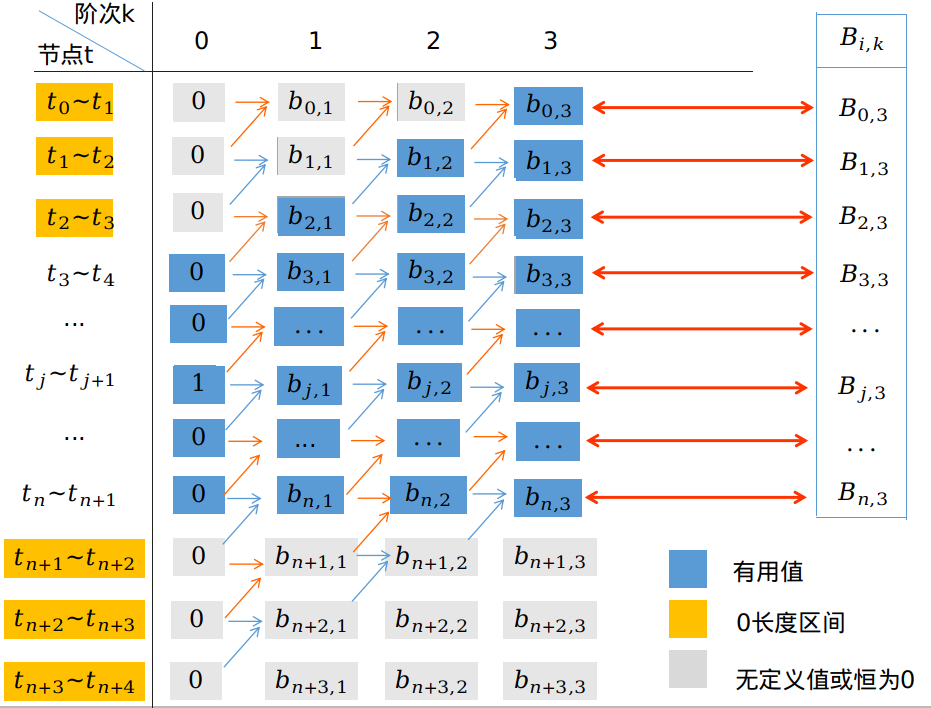注意，其中橙色标注的区间为 $[0,0] or [1,1]$ 长度为 0，另，又因为其不在定义域内，我们不作计算，也即，其 0 次 基函数值始终记为 0。假设我们计算定义域内某区间 $[t_j,t_{j+1}]$ 的曲线，按照前篇介绍的递推计算公式，0 次基函数 $b_{j,0} = 1$ 其余为 0 ，正如上表所示。
我们要得到 n+1 个点的权重，即 $B_{0,3},B_{1,3},\cdots,B_{n,3}$ ，由上我们知道，对于一段区间（如 $[t_j,t_{j+1}]$），三次基函数只会有四个非零值，分别为 $B_{j,3},B_{j-1,3},B_{j-2,3},B_{j-3,3}$
如前篇所述，递推公式为
$b_{j,k}(t) = \frac{t-t_j}{t_{j+k}-t_j}\ b_{j,k-1}(t)+\frac{t_{j+k+1}-t}{t_{j+k+1}-t_{j+1}}\ b_{j+1,k-1}(t)$
四个权重求解具体如下，当 $t\in[j,j+1]$

0 次 基函数有一个非零 $b_{j,0}$
$b_{j,0}=1$

1 次 基函数有两个非零 $b_{j,1},b_{j-1,1}$
\begin{aligned} b_{j,1}(t) & = \frac{t-t_j}{t_{j+1}-t_j}\ b_{j,0}(t)+\frac{t_{j+2}-t}{t_{j+2}-t_{j+1}}\ b_{j+1,0}(t) \\ & = \frac{t-t_j}{t_{j+1}-t_j} \end{aligned}
\begin{aligned} b_{j-1,1}(t) & = \frac{t-t_{j-1}}{t_{j}-t_{j-1}}\ b_{j-1,0}(t)+\frac{t_{j+1}-t}{t_{j+1}-t_{j}}\ b_{j,0}(t) \\ & = \frac{t_{j+1}-t}{t_{j+1}-t_{j}} \end{aligned}

2 次 基函数有三个非零 $b_{j,2},b_{j-1,2},b_{j-2,2}$
\begin{aligned} b_{j,2}(t) & = \frac{t-t_j}{t_{j+2}-t_j}\ b_{j,1}(t)+\frac{t_{j+3}-t}{t_{j+3}-t_{j+1}}\ b_{j+1,1}(t) \\ & = \frac{t-t_j}{t_{j+2}-t_j} \cdot \frac{t-t_j}{t_{j+1}-t_j} \\ & = \frac{(t-t_j)^2}{(t_{j+2}-t_j)(t_{j+1}-t_j)} \end{aligned}
\begin{aligned} b_{j-1,2}(t) & = \frac{t-t_{j-1}}{t_{j+1}-t_{j-1}}\ b_{j-1,1}(t)+\frac{t_{j+2}-t}{t_{j+2}-t_{j}}\ b_{j,1}(t) \\ & = \frac{t-t_{j-1}}{t_{j+1}-t_{j-1}}\cdot \frac{t_{j+1}-t}{t_{j+1}-t_{j}} +\frac{t_{j+2}-t}{t_{j+2}-t_{j}}\cdot \frac{t-t_j}{t_{j+1}-t_j} \\ & = \frac{(t-t_{j-1})(t_{j+1}-t)}{(t_{j+1}-t_{j-1})(t_{j+1}-t_{j})} + \frac{(t_{j+2}-t)(t-t_j)}{(t_{j+2}-t_{j})(t_{j+1}-t_j)} \end{aligned}
\begin{aligned} b_{j-2,2}(t) & = \frac{t-t_{j-2}}{t_{j}-t_{j-2}}\ b_{j-2,1}(t)+\frac{t_{j+1}-t}{t_{j+1}-t_{j-1}}\ b_{j-1,1}(t) \\ & = \frac{t_{j+1}-t}{t_{j+1}-t_{j-1}}\cdot \frac{t_{j+1}-t}{t_{j+1}-t_{j}} \\ & = \frac{(t_{j+1}-t)^2}{(t_{j+1}-t_{j-1})(t_{j+1}-t_{j})} \end{aligned}

3 次 基函数有四个非零 $b_{j,3},b_{j-1,3},b_{j-2,3},b_{j-3,3}$
\begin{aligned} b_{j,3}(t) &= \frac{t-t_j}{t_{j+3}-t_j}\ b_{j,2}(t)+\frac{t_{j+4}-t}{t_{j+4}-t_{j+1}}\ b_{j+1,2}(t) \\ & = \frac{t-t_j}{t_{j+3}-t_j}\cdot \frac{(t-t_j)^2}{(t_{j+2}-t_j)(t_{j+1}-t_j)} \\ & = \frac{(t-t_j)^3}{(t_{j+3}-t_j)(t_{j+2}-t_j)(t_{j+1}-t_j)} \end{aligned}
\begin{aligned} b_{j-1,3}(t) &= \frac{t-t_{j-1}}{t_{j+2}-t_{j-1}}\ b_{j-1,2}(t)+\frac{t_{j+3}-t}{t_{j+3}-t_{j}}\ b_{j,2}(t) \\ & = \frac{t-t_{j-1}}{t_{j+2}-t_{j-1}}\cdot [\frac{(t-t_{j-1})(t_{j+1}-t)}{(t_{j+1}-t_{j-1})(t_{j+1}-t_{j})} + \frac{(t_{j+2}-t)(t-t_j)}{(t_{j+2}-t_{j})(t_{j+1}-t_j)}] + \frac{t_{j+3}-t}{t_{j+3}-t_{j}}\cdot \frac{(t-t_j)^2}{(t_{j+2}-t_j)(t_{j+1}-t_j)} \\ & = \frac{(t-t_{j-1})^2(t_{j+1}-t)}{(t_{j+2}-t_{j-1})(t_{j+1}-t_{j-1})(t_{j+1}-t_{j})} + \frac{(t-t_{j-1})(t_{j+2}-t)(t-t_j)}{(t_{j+2}-t_{j-1})(t_{j+2}-t_{j})(t_{j+1}-t_j)} + \frac{(t_{j+3}-t)(t-t_j)^2}{(t_{j+3}-t_j)(t_{j+2}-t_j)(t_{j+1}-t_j)} \end{aligned}
\begin{aligned} b_{j-2,3}(t) &= \frac{t-t_{j-2}}{t_{j+1}-t_{j-2}}\ b_{j-2,2}(t)+\frac{t_{j+2}-t}{t_{j+2}-t_{j-1}}\ b_{j-1,2}(t) \\ & = \frac{t-t_{j-2}}{t_{j+1}-t_{j-2}}\cdot \frac{(t_{j+1}-t)(t_{j+1}-t)}{(t_{j+1}-t_{j-1})(t_{j+1}-t_{j})} + \frac{t_{j+2}-t}{t_{j+2}-t_{j-1}}\cdot [\frac{(t-t_{j-1})(t_{j+1}-t)}{(t_{j+1}-t_{j-1})(t_{j+1}-t_{j})} + \frac{(t_{j+2}-t)(t-t_j)}{(t_{j+2}-t_{j})(t_{j+1}-t_j)}] \\ & = \frac{(t-t_{j-2})(t_{j+1}-t)^2}{(t_{j+1}-t_{j-2})(t_{j+1}-t_{j-1})(t_{j+1}-t_{j})} + \frac{(t_{j+2}-t)(t-t_{j-1})(t_{j+1}-t)}{(t_{j+2}-t_{j-1})(t_{j+1}-t_{j-1})(t_{j+1}-t_{j})} + \frac{(t_{j+2}-t)^2(t-t_j)}{(t_{j+2}-t_{j-1})(t_{j+2}-t_{j})(t_{j+1}-t_j)} \end{aligned}
\begin{aligned} b_{j-3,3}(t) &= \frac{t-t_{j-3}}{t_{j}-t_{j-3}}\ b_{j-3,2}(t)+\frac{t_{j+1}-t}{t_{j+1}-t_{j-2}}\ b_{j-2,2}(t) \\ &= \frac{t_{j+1}-t}{t_{j+1}-t_{j-2}}\cdot \frac{(t_{j+1}-t)^2}{(t_{j+1}-t_{j-1})(t_{j+1}-t_{j})} \\ &= \frac{(t_{j+1}-t)^3}{(t_{j+1}-t_{j-2})(t_{j+1}-t_{j-1})(t_{j+1}-t_{j})} \end{aligned}

也即
\begin{aligned} &B_{j,3}(t) = \frac{(t-t_j)^3}{(t_{j+3}-t_j)(t_{j+2}-t_j)(t_{j+1}-t_j)} \\ &B_{j-1,3}(t) = \frac{(t-t_{j-1})^2(t_{j+1}-t)}{(t_{j+2}-t_{j-1})(t_{j+1}-t_{j-1})(t_{j+1}-t_{j})} + \frac{(t-t_{j-1})(t_{j+2}-t)(t-t_j)}{(t_{j+2}-t_{j-1})(t_{j+2}-t_{j})(t_{j+1}-t_j)} + \frac{(t_{j+3}-t)(t-t_j)^2}{(t_{j+3}-t_j)(t_{j+2}-t_j)(t_{j+1}-t_j)} \\ &B_{j-2,3}(t) = \frac{(t-t_{j-2})(t_{j+1}-t)^2}{(t_{j+1}-t_{j-2})(t_{j+1}-t_{j-1})(t_{j+1}-t_{j})} + \frac{(t_{j+2}-t)(t-t_{j-1})(t_{j+1}-t)}{(t_{j+2}-t_{j-1})(t_{j+1}-t_{j-1})(t_{j+1}-t_{j})} + \frac{(t_{j+2}-t)^2(t-t_j)}{(t_{j+2}-t_{j-1})(t_{j+2}-t_{j})(t_{j+1}-t_j)} \\ &B_{j-3,3}(t) = \frac{(t_{j+1}-t)^3}{(t_{j+1}-t_{j-2})(t_{j+1}-t_{j-1})(t_{j+1}-t_{j})} \end{aligned} \tag{*}
由此，得到该区间的曲线表达式为
\begin{aligned} B(t) & = B_{0,3}\ P_0 + B_{1,3}\ P_1 + \cdots + B_{n,3}\ P_n \\ & = B_{j-3,3}(t)\ P_{j-3} + B_{j-2,3}(t)\ P_{j-2} + B_{j-1,3}(t)\ P_{j-1} + B_{j,3}(t)\ P_j \end{aligned}
根据公式可知，当节点 $t_0,t_1,\cdots,t_n$ 确定后，$B_{j,3},B_{j-1,3},B_{j-2,3},B_{j-3,3}$ 都是关于 t 的三次函数。$B(t)$ 也随着 t $（\in[0,1]）$ 的取值而确定，也即确定了曲线上的一系列点 B(t)，正是这些点组成了样条曲线。
2.2.2 B样条插值方程的获取
clamped -B样条本身跟贝塞尔曲线很接近，当然可以考虑使用类似于上节贝塞尔曲线插值那样，在两点内依次插入 B 样条，通过某些设定确保曲线光滑。但这种方法会同上面一样很粗糙。
对于 B 样条，不同与贝塞尔曲线的一点就是，B 样条曲线有节点，而且节点可以映射到最终曲线上，节点对应坐标为 $B(t_j)$，依据以上公式计算。而且B样条本身就是一条光滑曲线，取代于上面贝塞尔曲线的两点间逐个插值，考虑使用B样条一次性生成所需曲线，且曲线通过原始点（型值点）。
而要满足生成的 B 样条直接穿过型值点，恰好可以利用上述节点性质（即节点在曲线上的有对应点 $B(t_j)$）。因此只需要考虑让 型值点等值于 节点对应点即可。即便这样，我们仍然有很大的参数设定自由度。

如，假设有一系列型值点中考虑其中一点 P’，我们使用三次B样条对所有型值点进行插值，让 P’ 等值于第 i 个节点对应点 $B(t_j)$ ，即
$P‘ = B(t_i) = B_{j-3,3}(t_j)\ P_{j-3} + B_{j-2,3}(t_j)\ P_{j-2} + B_{j-1,3}(t_j)\ P_{j-1} + B_{j,3}(t_j)\ P_j$
该式中，P‘ 已知，控制点 $P_{j-3},P_{j-2},P{j-1},P{j}$ 都未知，第 j 个节点值 $t_j$ 也未知，而且这些值都可以一定程度地"随意设置。
也就是说，我们有很大的自由度来通过手动设定控制点、节点分布等来满足上式。而只要满足上式，就会有生成的B样条曲线经过型值点 P’。
当然这是对一个型值点而言，考虑所有型值点的情况下，每个公式中的控制点会有重复，手动设定的自由度可能会下降，但大致感觉最终应该还是会有很多自由度。当然，可以用数学公式具体来计算说明，在此不赘述，仅为了定性说明问题。下面有详细分析求解过程。

同样以 三次B样条为例，假设已知有 l+1 个型值点为 $z_0,z_1,\cdots,z_l$ ；我们要插值生成的三次B样条参数有：$n+1$ 个控制点 $P_0,P_1,\cdots,P_n$  和 $m+1$ 个节点 $t_0,t_1,\cdots,t_m$ 。
首先需要明确的一点是，就目前来说，目标三次样条曲线中控制点个数没限制，节点数目没限制，仅有的一个限制是
$m = n + k + 1 = n + 4 \tag{1}$
对于每个没有限制的点（控制点、节点）都意味着一个个的自由度。下面开始施加限制
先考虑最重要的一点，即，使型值点与节点（确切说是，其在目标曲线上的点）对应，也即 $z_i = B(t_i)$。可以用如下图表示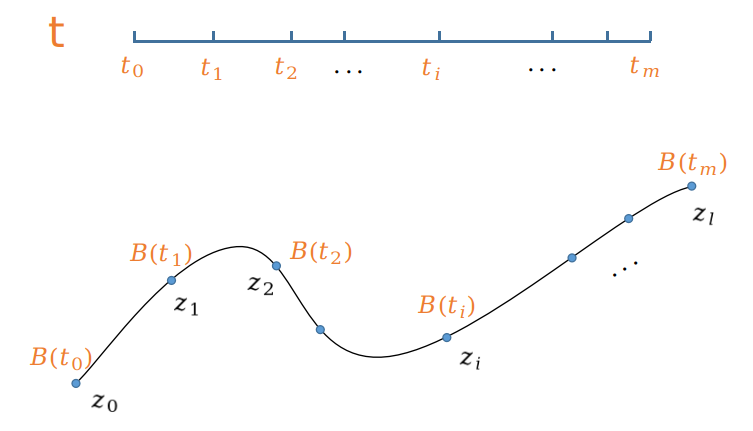上图中表示了，$z_0 = B(t_0), z_1=B(t_1), \cdots, z_n = B(t_m)$，此时是最简单的对应关系，此时有 $m=l$。但此时会有个问题，因为有一个不可忽视的一点，样条曲线是有定义域的，对于三次样条，定义域为 $[t_3,t_{n+1}]$，因此上述对应关系是有问题的，至少 $z_0$ 应该与定义域内第一个值 $B(t_3)$ 对应。同样，最后一个 $z_l$ 与 定义域内最后一个值 $B(t_{n+1})$ 对应。
上面提到了定义域的问题，一个极好的解决方案是使用 clamped B-spline
对于三次的 clamped B-样条，有
$t_0 = t_1 = t_2 = t_3 = 0 \\ t_4,t_5,\cdots,t_{n} \in[0,1] \\ t_{n+1} = t_{n+2} = t_{n+3} = t_{n+4} = 1$
而定义域为 $[t_3,t_{n+1}]$ ，因此我们采用的对应关系为
$t_3 \Leftrightarrow z_0 \\ t_5 \Leftrightarrow z_1 \\ \vdots \\ t_{n+1} \Leftrightarrow z_l$
也即有
$z_0 = B(t_3) = B(0) \\ \vdots \\ z_j = B(t_{j+3}) \\ \vdots \\ z_l = B(t_{n+1})=B(1) \tag{2}$
此时，$n+1 = l+3$ 即满足 $n = l+2$。
综上所述，根据限制，我们需要满足的关系有：

需要设定样条的控制点的数目比型值点多2个，即
$n = l + 2$

需要设定的节点数目比型值点多6个，即
$m = n + k + 1 = l+2+3+1=l+6$

满足一系列关系式（共 $l+1$ 个）
$z_{j-3} = B(t_j) =B_{j-3,3}(t_j)\ P_{j-3} + B_{j-2,3}(t_j)\ P_{j-2} + B_{j-1,3}(t_j)\ P_{j-1} + B_{j,3}(t_j)\ P_j\ ,\ \ \ j = 3,4,\cdots,l+3$
且对于 $B_{j,3},B_{j-1,3},B_{j-2,3},B_{j-3,3}$ ，带入（*）公式得
\begin{aligned} &B_{j-3,3}(t_j) = \frac{(t_{j+1}-t_j)^2}{(t_{j+1}-t_{j-2})(t_{j+1}-t_{j-1})} \\ &B_{j-2,3}(t_j) = \frac{(t_j-t_{j-2})(t_{j+1}-t_j)^2}{(t_{j+1}-t_{j-2})(t_{j+1}-t_{j-1})(t_{j+1}-t_{j})} + \frac{(t_{j+2}-t_j)(t_j-t_{j-1})}{(t_{j+2}-t_{j-1})(t_{j+1}-t_{j-1})} \\ &B_{j-1,3}(t_j) = \frac{(t_j-t_{j-1})^2(t_{j+1}-t_j)}{(t_{j+2}-t_{j-1})(t_{j+1}-t_{j-1})(t_{j+1}-t_{j})} \\ &B_{j,3}(t_j) = \frac{(t-t_j)^3}{(t_{j+3}-t_j)(t_{j+2}-t_j)(t_{j+1}-t_j)} = 0\\ \end{aligned}
式子 $B_{j,3} = 0$ ，当 t 列表确定时，$B_{j-1,3},B_{j-2,3},B_{j-3,3}$ 都为定值。上述关系式变为
$z_{j-3} = B(t_j) =B_{j-3,3}(t_j)\ P_{j-3} + B_{j-2,3}(t_j)\ P_{j-2} + B_{j-1,3}(t_j)\ P_{j-1},\ \ \ j = 3,4,\cdots,l+3$
展开
$z_0 = B_{0,3}(t_3)\ P_{0} + B_{1,3}(t_3)\ P_{1} + B_{2,3}(t_3)\ P_2 \\ z_1 = B_{1,3}(t_4)\ P_{1} + B_{2,3}(t_4)\ P_{2} + B_{3,3}(t_4)\ P_3 \\ \vdots \\ z_l = B_{l,3}(t_{l+3})\ P_{l} + B_{l+1,3}(t_{l+3}\ P_{l+1} + B_{l+2,3}(t_{l+3})\ P_{l+2}$
用矩阵更简洁地表示为
$\left[ \begin{matrix} z_0 \\ z_1 \\ \vdots \\ z_{l} \end{matrix} \right] =\left[ \begin{matrix} B_{0,3}(t_3) & B_{1,3}(t_3) & B_{2,3}(t_3) & 0 & \cdots & 0\\ 0 & B_{1,3}(t_4) & B_{2,3}(t_4) & B_{3,3}(t_4) & \cdots & 0\\ \vdots & 0 & \ddots & & & 0 \\ 0 & \cdots & 0 & B_{l,3}(t_{l+3}) & B_{l+1,3}(t_{l+3}) & B_{l+2,3}(t_{l+3}) \end{matrix} \right] \left[ \begin{matrix} P_0 \\ P_1 \\ \vdots \\ P_{l+2} \end{matrix} \right]$

且已知
$t_0 = t_1 = t_2 = t_3 = 0 \\ t_{l+3} = t_{l+4} = t_{l+5} = t_{l+6} = 1$
则可得
\begin{aligned} &B_{0,3}(t_3) = \frac{(t_{4}-t_3)^2}{(t_{4}-t_{1})(t_{4}-t_{2})} = 1 \\ &B_{1,3}(t_3) = \frac{(t_3-t_{1})(t_{4}-t_3)^2}{(t_{4}-t_{1})(t_{4}-t_{2})(t_{4}-t_{3})} + \frac{(t_{5}-t_3)(t_3-t_{2})}{(t_{5}-t_{2})(t_{4}-t_{2})} = 0 \\ &B_{2,3}(t_3) = \frac{(t_3-t_{2})^2(t_{4}-t_3)}{(t_{5}-t_{2})(t_{4}-t_{2})(t_{4}-t_{3})} = 0 \end{aligned}
那么，对于方程组第一个式子，就变为
$z_0 = B_{0,3}(t_3)\ P_{0} + B_{1,3}(t_3)\ P_{1} + B_{2,3}(t_3)\ P_2 = P_0$